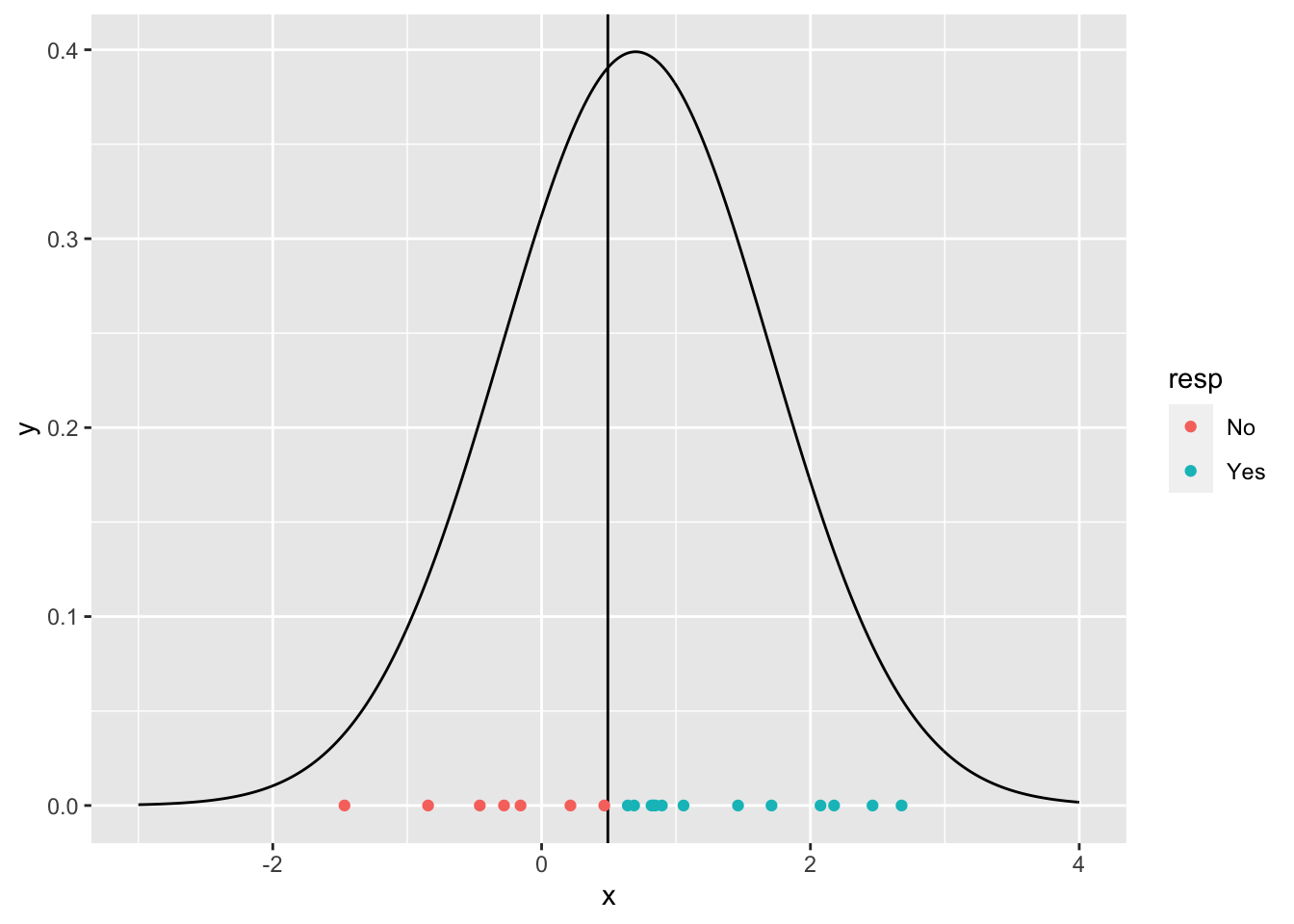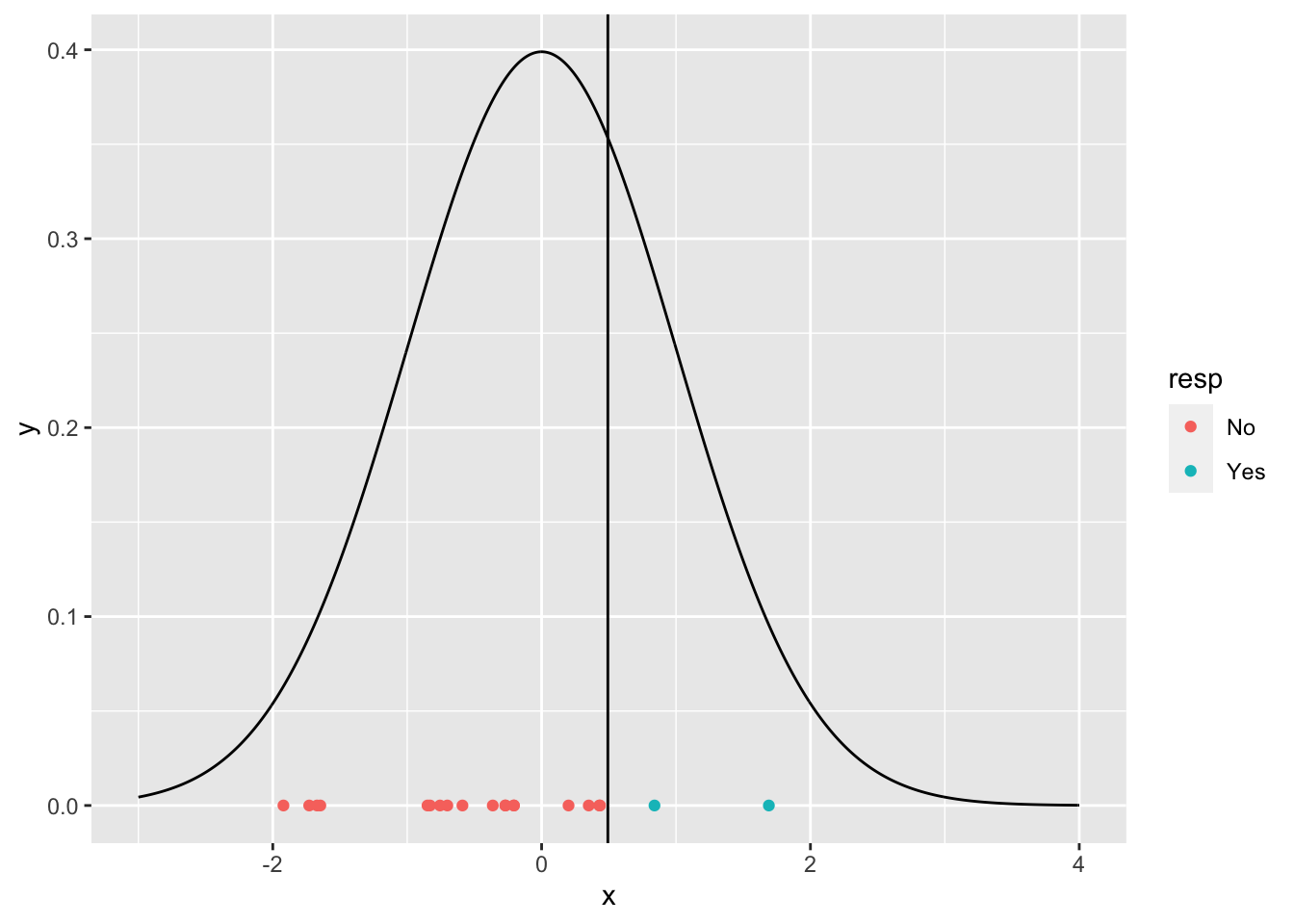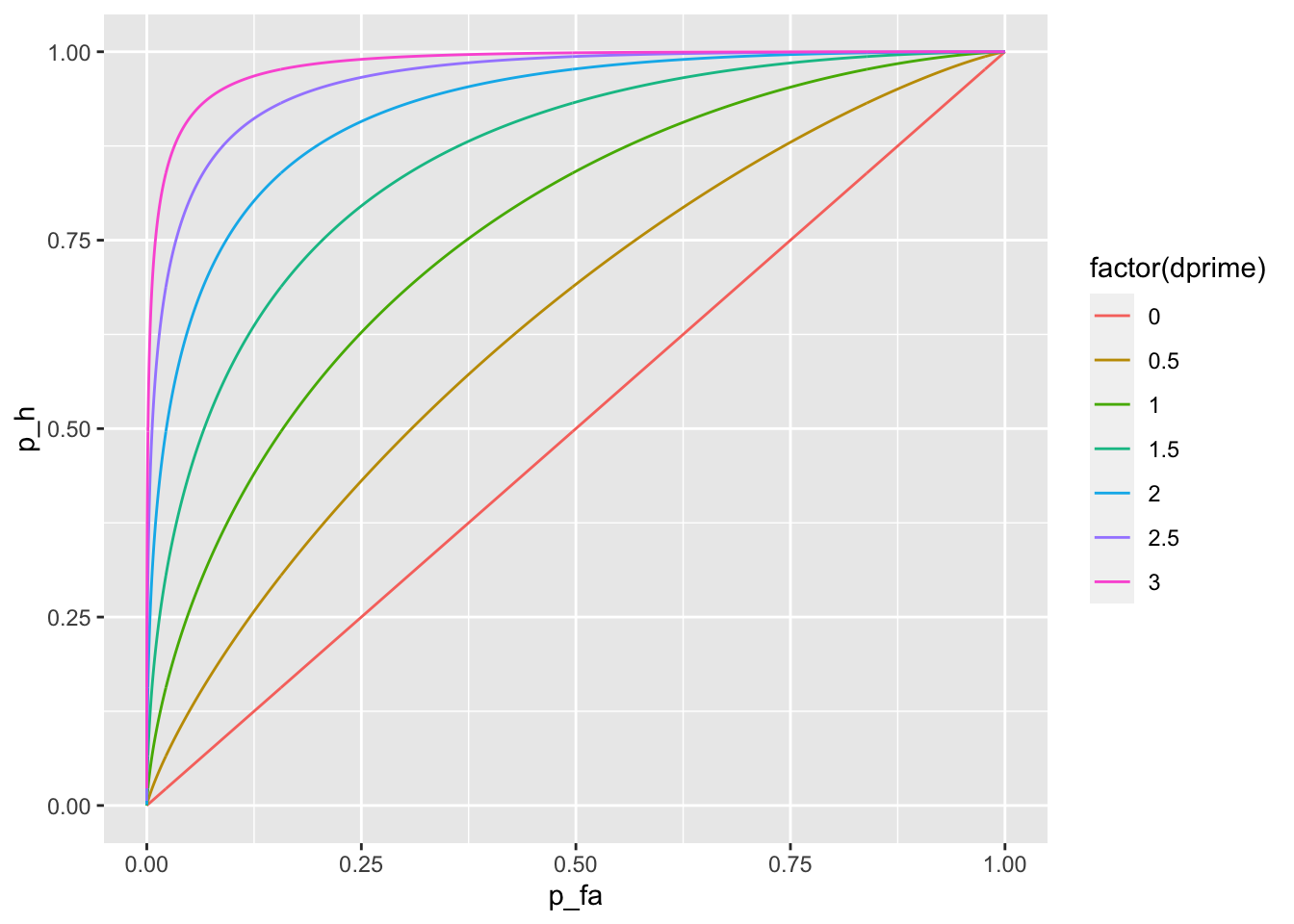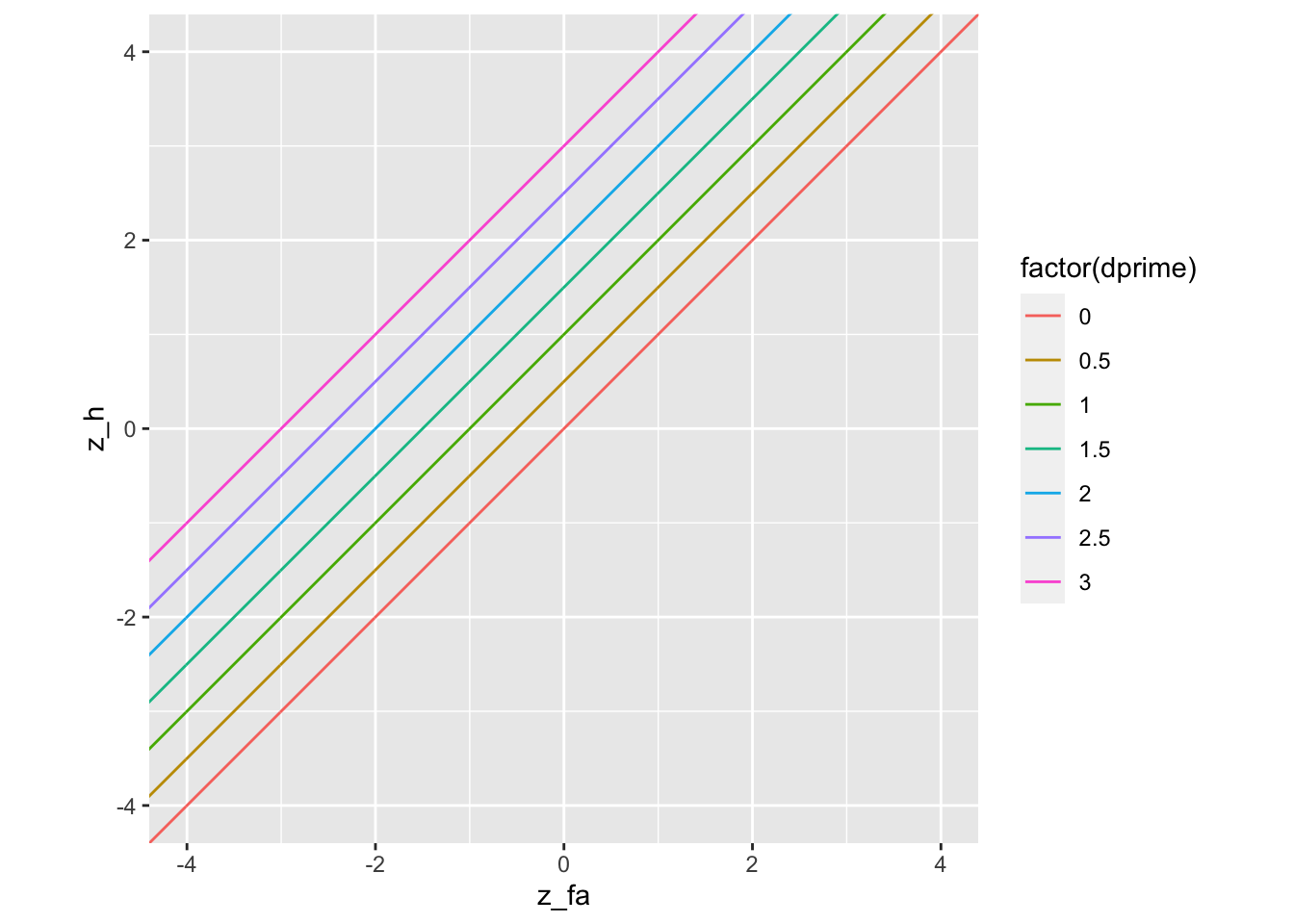## Assumed distributions of signal and noise

$$X_n \sim N(0,1) =f_n(x | \theta=0)= \frac{1}{\sqrt{2\pi}}e^{-\frac{x^2}{2}}$$

$$X_s \sim N(d',1) =f_s(x | \theta=d')= \frac{1}{\sqrt{2\pi}}e^{-\frac{(x-d')^2}{2}}$$

## Likelihood

The likelihood is defined as (in SDT it is as the inverse of the the likelihood ratio most often used in statistics). $\Lambda (x) = \frac{f_S(x)}{f_N(x)}$

It can be demonstrated similarly to here that

$\log{\Lambda}(x) = d' \left( x - d' / 2\right)$

## Decision rule

If $$\log{\Lambda} > \lambda$$ choose $$a_S$$

which results in

$\text{If }x > c =\frac{\lambda}{d'} + \frac{d'}{2} \text{ then }a=a_s$ $\text{If }x < c =\frac{\lambda}{d'} + \frac{d'}{2} \text{ then }a=a_n$

That is, the criterium can be imposed direclty in the sample from the random variable. The observer model is completely specified by $$d'$$ and $$\lambda$$.

### Example

Trials with the signal

library(tidyverse)

dprime <- .7
lambda <- .1
crit <- lambda / dprime + .5 *dprime

dat_s <- tibble(trial = 1:20) %>%
mutate(x = rnorm(n(), dprime, 1),
resp = if_else(x > crit, "Yes", "No"))
dat_s
## # A tibble: 20 x 3
##    trial      x resp
##    <int>  <dbl> <chr>
##  1     1  0.832 Yes
##  2     2  2.46  Yes
##  3     3 -0.280 No
##  4     4 -0.845 No
##  5     5  0.214 No
##  6     6  0.688 Yes
##  7     7  0.641 Yes
##  8     8  1.71  Yes
##  9     9  0.895 Yes
## 10    10  1.06  Yes
## 11    11 -1.47  No
## 12    12  2.18  Yes
## 13    13  0.467 No
## 14    14  2.08  Yes
## 15    15  1.46  Yes
## 16    16 -0.157 No
## 17    17  0.818 Yes
## 18    18  0.846 Yes
## 19    19  2.68  Yes
## 20    20 -0.460 No
ggplot(dat_s) +
geom_line(data = tibble(x = seq(-3, 4, 0.01),
y = dnorm(x, dprime, 1)),
aes(x = x, y = y)) +
geom_vline(xintercept = crit) +
geom_point(aes(x = x, y = 0, color = resp))For the noise trials

dat_n <- tibble(trial = 1:20) %>%
mutate(x = rnorm(n(), 0, 1),
resp = if_else(x > crit, "Yes", "No"))
dat_n
## # A tibble: 20 x 3
##    trial      x resp
##    <int>  <dbl> <chr>
##  1     1 -0.206 No
##  2     2 -0.363 No
##  3     3 -1.65  No
##  4     4 -0.849 No
##  5     5  1.69  Yes
##  6     6 -1.92  No
##  7     7  0.200 No
##  8     8 -1.67  No
##  9     9 -0.701 No
## 10    10  0.840 Yes
## 11    11 -0.589 No
## 12    12  0.351 No
## 13    13 -0.206 No
## 14    14 -0.269 No
## 15    15 -0.270 No
## 16    16 -0.830 No
## 17    17  0.434 No
## 18    18 -1.73  No
## 19    19 -0.756 No
## 20    20  0.429 No
ggplot(dat_n) +
geom_line(data = tibble(x = seq(-3, 4, 0.01),
y = dnorm(x, 0, 1)),
aes(x = x, y = y)) +
geom_vline(xintercept = crit) +
geom_point(aes(x = x, y = 0, color = resp))## Probabilities

Under the 0-1 loss model, the observer model can be reparametrized in terms of the probabilities

$$p_{FA}=P_{\mu=0}\left(d(X)=a_s\right) = P_{\mu=0}\left(x > c\right) = 1 - \Phi(c)$$

$$p_{H}=P_{\mu=d'}\left(d(X)=a_s\right) = P_{\mu=d'}\left(x > c\right) = 1 - \Phi(c-d')$$

then

$$c = \Phi^{-1} (1 -p_{FA})=- \Phi^{-1} (p_{FA})$$

$$d' = c - \Phi^{-1} (1 -p_{H})= \Phi^{-1} (p_{H}) - \Phi^{-1} (p_{FA})$$

## Other parametrizations of the criterion

Sometimes, a criterion measured from the unbiased criterion is used

$$c_{center} = c - \frac{d'}{2} = - \frac{1}{2} \left( \Phi^{-1} (p_{H}) + \Phi^{-1} (p_{FA}) \right)$$

Another popular criterion is the relative heights of the distributions at $$c$$

$$\beta = \Lambda(c)$$

Another criterion used in $$\lambda$$, often called bias, which expressed in terms of $$c$$ is

$\lambda = d' \left( c - \frac{d'}{2} \right)$ and in terms of $$c_{center}$$ is

$\lambda = d' c_{center}$ and in terms of proportion of hits and false alarms is

$\lambda = \frac{1}{2} \left( (\Phi^{-1}(p_{FA}))^2 - (\Phi^{-1}(p_{H}))^2\right)$

## Optimal performance

From here

$c^{*} =\frac{\lambda^{*}}{d'} + \frac{d'}{2} =\frac{-\text{logit}(P(H_S))}{d'} + \frac{d'}{2}$

If $$P(H_S) = 1/2$$, then

$c^{*} = \frac{d'}{2}$

## Parameter estimation

The MLE of $$p_{FA}$$ is $$\widehat{p}_{FA}=\frac{n_{FA}}{ n_{FA} + n_{CR}}$$.

The MLE of $$p_{H}$$ is $$\widehat{p}_{H}=\frac{n_{H}}{ n_{H} + n_{M}}$$.

Given that $$(d',c)$$ is an invertible transformation form $$(p_H,p_{FA})$$, we can calculate $$\widehat{d}'$$ and $$\widehat{c}$$ from $$\widehat{p}_{H}$$ and $$\widehat{p}_{FA}$$.

## ROC curves

If the observer model is the equal-variance model, the ROC curve for an observer with fixed sensitivity will be calculated as

$$p_{FA} = 1 - \Phi(c)$$

$$p_{H} = 1 - \Phi(c-d')$$

Example of several ROC curves for different sensitivities

dprime <- seq(0, 3, .5)
crit <- seq(-5, 5, .01)

crossing(dprime, crit) %>%
mutate(p_fa = 1 - pnorm(crit),
p_h = 1 - pnorm(crit - dprime)) %>%
ggplot(aes(x = p_fa, y = p_h, color = factor(dprime))) +
geom_line() +
xlim(0, 1) +
ylim(0, 1)### zROC

Given

$$p_{FA} = 1 - \Phi(c) = \Phi(-c)$$

$$p_{H} = 1 - \Phi(c-d') = \Phi(d'-c)$$

then

$$\Phi^{-1}(p_{FA}) = -c$$

$$\Phi^{-1}(p_{H}) = d'-c$$

That is

$$\Phi^{-1}(p_{H}) = d' + \Phi^{-1}(p_{FA})$$

The slope is 1 and the intercept is $$d'$$.

crossing(dprime, crit) %>%
mutate(z_fa = -crit,
z_h = dprime - crit) %>%
ggplot(aes(x = z_fa, y = z_h, color = factor(dprime))) +
geom_line() +
coord_equal(xlim = c(-4, 4), ylim = c(-4, 4))